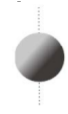# Angular momentum – problems and solutions

1. An object with the moment of inertia of 2 kg m2 rotates at 1 rad/s. What is the angular momentum of the object?

Known :

Moment of inertia (I) = 2 kg m2

Angular speed (ω) = 1 rad/s

Wanted : Angular momentum (L)

Solution :

Formula of angular momentum :

L = I ω

L = angular momentum (kg m2/s), I = moment of inertia (kg m2), ω = angular speed (rad/s)

The angular momentum :

L = I ω = (2)(1) = 2 kg m2/s

[irp]

2. A 2-kg cylinder pulley with radius of 0.1 m rotates at a constant angular speed of 2 rad/s. What is the angular momentum of the pulley ?Known :

Mass of pulley (m) = 2 kg

Radius of pulley (r) = 0.1 m

Angular speed (ω) = 2 rad/s

Wanted : Angular momentum

Solution :

Formula of moment of inertia for solid cylinder :

I = 1/2 m r2

I = moment of inertia (kg m2), m = mass (kg), r = radius (m)

Moment of inertia :

I = 1/2 (2)(0.1)2 = (1)(0.01) = 0.01 kg m2

The angular speed :

L = I ω = (0.01)(2) = 0.02 kg m2/s

[irp]

3. A 2-kg uniform sphere with radius of 0.2 m rotates at 4 rad/s. What is the angular momentum of the ball.Known :

Mass of ball (m) = 2 kg

Radius of ball (r) = 0.2 m

Angular speed (ω) = 4 rad/s

Wanted : Angular momentum

Solution :

Formula of moment of inertia for uniform sphere :

I = (2/5) m r2

I = moment of inertia (kg m2), m = mass (kg), r = radius (m)

The moment of inertia for uniform sphere :

I = (2/5)(2)(0.2)2 = (4/5)(0.04) = 0.032 kg m2

The angular momentum of sphere :

L = I ω = (0.032)(4) = 0.128 kg m2/s

[irp]

4. A 1-kg particle rotates at a constant angular speed of 2 rad/s. What is the angular speed if the radius of circle is 10 cm.

Known :

Mass of object (m) = 1 kg

The radius of circle (r) = 10 cm = 10/100 = 0.1 m

The angular speed (ω) = 2 rad/s

Wanted : Angular momentum

Solution :

Formula of moment of inertia for particle :

I = m r2 = (1)(0.1)2 = (1)(0.01) = 0.01 kg m2

Angular momentum :

L = I ω = (0.01)(2) = 0.02 kg m2/s